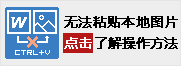•  您的网站试用资格已过期。

# 【信息会考】python基础知识

python基础知识

1. 计算机程序解决问题的基本过程应该是下列哪个选项。（）

 A．①②③④ B．③①④②(正确答案) C．①④②③ D．④③②①

2. 以下描述中最适合用计算机编程来处理的是（）

 A．确定放学回家的路线 B．处理一张数码照片 C．计算10000以内的奇数和(正确答案) D．在因特网上查找歌曲

3. 将两个变量互换的算法描述如图所示，描述的方式是（）A．伪代码 B．流程图 C．PAD图 D．自然语言(正确答案)

4. Python文件的后缀名是（）。

 A．.doc B．.vbp C．.py(正确答案) D．.exe

5. 如图所示的流程图中，红色虚线框内结束应该使用的基本图形是（）。A．① B．②(正确答案) C．③ D．④

6. python IDLE中，字符串的颜色为

 A．橘色 B．紫色 C．绿色(正确答案) D．红色

7. python中，#号的作用是

 A．电话号码 B．颜色代码 C．注释代码(正确答案) D．代码断点

8. 以下是一段python程序:它的可以用哪个结构表达（）。A．顺序结构 B．分支结构(正确答案) C．循环结构

9. 高级语言更接近自然语言，并不特指某种语言;也不依赖特定的计算机系统，因而更容易掌握和使用，通用性也更好。以下不属于高级语言的是（）

 A．Java语言 B．Python语言 C．汇编语言(正确答案) D．VB语言

10. 描述算法可以有多种方法，以下不是算法描述的方法（）。

 A．流程图 B．程序代码 C．自然语言 D．树形图(正确答案)

11. 下列的变量名称合法的是（）

 A．1he B．he_1(正确答案) C．he&1 D．import

12. python表达式 9%2 +10**2 // 6的结果是（）

 A．True B．17(正确答案) C．7 D．20.6

13. 若变量a = 5b = 4c = 3，则表达式(a != b) and ( b < c)的值是（）。

 A．True B．Flase(正确答案) C．4 D．无解

14. 下列赋值语句正确的是（）

 A．x+1=x B．x=x+1(正确答案) C．x=+1 D．x,y,z=1,2

15. Python IDLE编辑器中书写正确的表达式（）

 A．b**2-4ac B．1/2gt2 C．pi*r^2 D．2*pi*r(正确答案)

16. str（）属于的数据类型（）

 A．字符型(正确答案) B．整型 C．浮点型 D．A,B,C全错

17. 下列不可以将变量xy的值互换的语句（）

 A．z=x;x=y;y=z B．x,y=y,x C．x=y;y=x(正确答案) D．A,B,C都可以互换。

18. Python3中，请问下列的输出语句正确的是：( )

 A．x=print(x) B．print(x)(正确答案) C．print x D．x print (x)

19. 请问通过输入函数输进的内容属于什么类型？( )

 A．字符串(正确答案) B．数字型 C．布尔型 D．文件型

20. 条件bmi18.524之间如何书写：(错误的是)

 A．bmi>18.5 or bmi<24(正确答案) B．18.518.5 and bmi<24 D．24>bmi>18.5

21. 以下表达式值为3的是（

 A．x==2*2-1 B．10%7(正确答案) C．43//10 D．10/3<3

22. 阅读程序，请问结果是（
a,b=2,3
c=a
a=b
b=c
print(a,b,c)

 A．2 3 3 B．3 2 2(正确答案) C．2 3 2 D．2 2 3

a=b       a

b=c       b

23. 判断闰年程序正确的是()

 A．y%400==0 or (y%100!=0 and y%4==0)(正确答案) B．y%400 and (y%100!=0 and y%4==0) C．y%400 or (y%100 or y%4==0) D．y%400==0 or (y%100!=0 or y%4==0)

24. 下列程序运行出现错误，你认为出错的原因是什么？()
guess="888"
x=input("请输入密码")
if x=guess:
print("你猜对了")
else:
print("你猜错了")

 A．输入函数书写错误 B．数据类型没有转换 C．判断表达式书写错误(正确答案) D．缺少elif语句

25. 程序功能为输入月份，判断是否为30天，以下程序是否正确：
m = int(input("请输入月份"))
if m==4 or 6 or 9 or 11:
print("30")

 A．正确 B．错误(正确答案) C．看运气 D．不知道

26. 阅读程序，请问结果是（
a=""

b="写诗"
a=a*3+"

print(a,b)

_________________________________(答案：我我我也会写诗)

27. 比较两数的大小，输出较大的数,填空为（
a = float(input("请输入a="))

b =float(input("请输入b="))
if ________:
print (a)
else:
print (b)

_________________________________(答案：ab a=bba|b=a)

28.. 根据福州市的士公司规则，首3公里打车费是12元，超过3公里每公里加收2.6元，请在①②③位置填空,用空格分隔开，例：① ② ③

k =float(input("请输入公里数："))
if _________
_________ :
f = _____
___________
else:
f = 12 + (k - 3) * 2.6
print ("